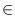Next: Circles ( circle ) Up: Basic Data Types for Previous: Straight Rays ( ray   Contents   Index

# Straight Lines ( line )

Definition

An instance l of the data type line is a directed straight line in the two-dimensional plane. The angle between a right oriented horizontal line and l is called the direction of l.

#include < LEDA/geo/line.h >

Types

 line::coord_type the coordinate type (double). line::point_type the point type (point).

Creation

 line l(const point& p, const point& q) introduces a variable l of type line. l is initialized to the line passing through points p and q directed form p to q. line l(const segment& s) introduces a variable l of type line. l is initialized to the line supporting segment s. line l(const ray& r) introduces a variable l of type line. l is initialized to the line supporting ray r. line l(const point& p, const vector& v) introduces a variable l of type line. l is initialized to the line passing through points p and p + v. line l(const point& p, double alpha) introduces a variable l of type line. l is initialized to the line passing through point p with direction alpha. line l introduces a variable l of type line. l is initialized to the line passing through the origin with direction 0.

Operations

 point l.point1() returns a point on l. point l.point2() returns a second point on l. segment l.seg() returns a segment on l. double l.angle(const line& g) returns the angle between l and g, i.e., g.direction() - l.direction(). double l.direction() returns the direction of l. double l.angle() returns l.direction(). bool l.is_vertical() returns true iff l is vertical. bool l.is_horizontal() returns true iff l is horizontal. double l.sqr_dist(const point& q) returns the square of the distance between l and q. double l.distance(const point& q) returns the distance between l and q. int l.orientation(const point& p) returns orientation(l.point1(), l.point2(), p). double l.slope() returns the slope of l. Precondition l is not vertical. double l.y_proj(double x) returns p.ycoord(), where pl with p.xcoord() = x. Precondition l is not vertical. double l.x_proj(double y) returns p.xcoord(), where pl with p.ycoord() = y. Precondition l is not horizontal. double l.y_abs() returns the y-abscissa of l (l.y_proj(0)). Precondition l is not vertical. bool l.intersection(const line& g, point& p) if l and g intersect in a single point this point is assigned to p and the result is true, otherwise the result is false. bool l.intersection(const segment& s, point& inter) if l and s intersect in a single point this point is assigned to p and the result is true, otherwise the result is false. bool l.intersection(const segment& s) returns true, if l and s intersect, false otherwise. line l.translate_by_angle(double a, double d) returns l translated in direction a by distance d. line l.translate(double dx, double dy) returns l translated by vector (dx, dy). line l.translate(const vector& v) returns l translated by vector v. Precondition v.dim() = 2. line l + const vector& v returns l translated by vector v. line l - const vector& v returns l translated by vector - v. line l.rotate(const point& q, double a) returns l rotated about point q by angle a. line l.rotate(double a) returns l.rotate( point(0, 0), a). line l.rotate90(const point& q, int i=1) returns l rotated about q by an angle of i x 90 degrees. If i > 0 the rotation is counter-clockwise otherwise it is clockwise. line l.reflect(const point& p, const point& q) returns l reflected across the straight line passing through p and q. line l.reverse() returns l reversed. segment l.perpendicular(const point& p) returns the segment perpendicular to l with source p. and target on l. point l.dual() returns the point dual to l. Precondition l is not vertical. int l.side_of(const point& p) computes orientation(a, b, p), where ab and a and b appear in this order on line l. bool l.contains(const point& p) returns true if p lies on l. bool l.clip(point p, point q, segment& s) clips l at the rectangle R defined by p and q. Returns true if the intersection of R and l is non-empty and returns false otherwise. If the intersection is non-empty the intersection is assigned to s; It is guaranteed that the source node of s is no larger than its target node.

Non-Member Functions

 int orientation(const line& l, const point& p) computes orientation(a, b, p), where ab and a and b appear in this order on line l. int cmp_slopes(const line& l1, const line& l2) returns compare(slope(l1), slope(l2)).Next: Circles ( circle ) Up: Basic Data Types for Previous: Straight Rays ( ray   Contents   Index